## Priya’s cat weights 5 1/2 pounds and her dog weighs 8 1/4 pounds. The dog is ______ pounds heavier than the cat.

Question

Priya’s cat weights 5 1/2 pounds and her dog weighs 8 1/4 pounds. The dog is ______ pounds heavier than the cat.

in progress 0
6 months 2021-08-07T16:09:02+00:00 2 Answers 11 views 0

1. 2 3/4

Steps

8 1/4 – 5 1/2

a. Find Common Denominator

8 1/4 – 5 2/4

b. Make Improper Fractions

8 × 4 + 1 = 33

33/4

5 × 4 + 2

22/4

c. Subtract

33/4 – 22/4 = 11/4

d. Simplify

11/4 = 2 3/4

Therefore, Priya’s dog is 2 3/4 pounds heavier than the cat.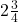Step-by-step explanation:

We have to subtract the weight of the cat from the weight of the dog.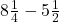The first issue here is that there is not a common denominator. I’m going to multiply that 1/2 by 2 to make it 2/4. The 5 does NOT change.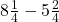Subtract the whole numbers first.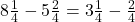Then, we’ll convert the first term to an improper fraction. We can do that by multiplying the whole number by the denominator and adding that number to the numerator.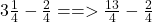Solve.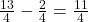Then, convert to a mixed number by dividing 11 by 4 and leaving the remainder in the numerator and putting the quotient in front as the whole number.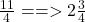So, the answer is